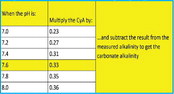Posted on

# 100 – (.33 x 90) ….100 – (.33 x 90) = 70 It is important to note that the one third correction factor is pH dependent. That is because the cyanuric acid and cyanurate ion concentrations are pH dependent. Therefore, it is best to use correction factors that account for this pH dependency.

The cyanuric acid correction factors may be found in the accompanying table. Borate Correction

The borate correction factor is pH dependent. The borate correction factors may be found in the accompanying table.

To obtain the carbonate alkalinity from the measured total alkalinity in the presence of borates, the following procedure can be used.

1. Select a borate factor based on the measured pH: F(B) 2. Calculate the carbonate alkalinity from the measured total alkalinity, the measured borate concentration (B), and

the selected borate factor (F(B))

Alkalinity corrected = Alkalinity total – (B x F(B))

For example, if the measured total alkalinity is 100 ppm, the pH is 7.6, and the measured borate concentration is 50 ppm, then: Alkalinity corrected = 100 – (50 x 0.17) Alkalinity corrected = 92 ppm

Putting it all together

As will soon become apparent, calculating the LSI in the presence of cyanuric acid and borates is a bit complicated, which is why many of today’s pool service techs simply install smart phone LSI calculators to save time.

However, for those interested in learning how to calculate the saturation index by hand, the following formula is applied to the water balance parameters: SI = pH + F(T) + F(TA) + F(CH) – F(TDS)

However, a calculated carbonate alkalinity must be used prior to selecting F(TA) For example, consider the following measured water parameters pH = 7.6; Temperature = 76; Total Alkalinity = 100 ppm; Cyanuric Acid = 90 ppm; Borates = 50 ppm; Calcium Hardness = 200 ppm; Total Dissolved Solids = 800 ppm First, calculate the carbonate alkalinity, or Alkalinity corrected: The Alkalinity corrected is obtained using the cyanuric acid and borate correction factors and the following formula:

Alkalinity corrected = TA – (CYA X .33) – (B x F(B))

Alkalinity corrected = 100 – (100 x .33) – (50 x 0.17) Alkalinity corrected = 58.5 ppm Next, find the factors for the Langelier Saturation Index from the accompanying table and simply plug them into the general formula:

LSI = pH + F(T) + F(TA) + F(CH) – F(TDS)

LSI = 7.6 + 0.6 + 1.8 + 1.9- 12.1 LSI = -0.2 The LSI is within range.

Cyanuric Acid Correction factors as a function of pH

Borate Correction Factors as a function of pHLATEST NEWS